# Improved Aziz Prediction Model of Pressure Gradient for Multiphase Flow in Wells

Improved Aziz Prediction Model of Pressure Gradient for Multiphase Flow in Wells

Mengxia Li Ruiquan Liao Wei Luo Yong Dong*

School of Information and Mathematics, Yangtze University, Jingzhou Hubei 434023, China

Petroleum Engineering College, Yangtze University, Wuhan Hubei 430100, China

The Branch of Key Laboratory of CNPC for Oil and Gas Production, Yangtze University, Wuhan Hubei 430100, China

School of Geosciences, Yangtze University, Wuhan Hubei 430100, China

Corresponding Author Email:
dongyong80@126.com
Page:
423-428
|
DOI:
https://doi.org/10.18280/ijht.340311
|
Accepted:
|
Published:
30 September 2016
| Citation

OPEN ACCESS

Abstract:

It is crucial for the completion parameter design and production performance detection interpretation to have an accurate pressure gradient. The Aziz prediction model of pressure gradient is a common calculation model in oil-gas field development. The laboratory experiment results of multiphase flow show that the average prediction relative error is 29.62% and the maximum relative error reaches 70.1%. By comparing the prediction residual of the Aziz model with the experiment condition parameters, as the volume flow rate of the liquid phase is constant, this paper considers that the prediction residual of the Aziz model is closely related to the gas liquid ratio and has no clear correlation with the water fraction of fluid phase. Based on unifying the orders of magnitude of the prediction residual and the gas liquid ratio, this paper uses a cubic function of the gas liquid ratio to fit the prediction residual of the model to obtain a new pressure prediction method which is called Aziz-I model. The results for ninety groups of experimental data show that the average prediction relative error of the Aziz-I model is reduced to 10.82%. Hence, the Aziz-I model improves the prediction accuracy of pressure gradient for multiphase flow.

Keywords:

Pressure gradient, Multiphase flow, Prediction, Aziz model, Function fitting.

1. Introduction

Having an accurate prediction of the pressure gradient of the multiphase flow in oil and gas wells is essential for the completion parameter design and production performance detection interpretation. The pressure gradient prediction is fundamental in the process of petroleum reservoir exploitation. It determines many aspects in the dynamically changing process of petroleum reservoir exploitation. Many researchers have done much work [1-5] in this field.

Internationally, academics have long been working on the problem of multiphase flow in wells, having proposed several prediction models. Without considering the models that have not received wide acceptance, among the proposed models are the common models of the Hagedorn-Brown model, Orkiszewski model, Aziz model, Beggs-Brill model, Mukherjee-Brill model, Hasan model, Ansari model, Kaya model, Cheng Jialiang model, Wang qisheng model, Liao model. These models are empirical or semi-empirical correlations. Although there are many continued improvements on the pressure gradient prediction models by some researchers, statistical calculation shows that they are not very satisfactory in obtaining the pressure gradient currently. On one hand, because of the complexity of the multiphase flow, it is difficult to find an accurate model, and the model used is only approximate for describing some parameters, resulting in model error. On the other hand, there are many variable factors in collecting data, resulting in observation error. Hence, these errors affect the prediction results.

In recent years, artificial intelligence methods (neural network, fuzzy logic, genetic algorithm, etc.) have become an increasingly powerful and reliable technological means for petroleum engineers to analyze and interpret the problems in oil and gas production areas. In 2004, Ayoub  proposed the common pressure drop model in pipes by using the neural network method. In 2010, Mohsen Ebrahimi  introduced the fuzzy logic and neural network for the research of the pressure drop prediction models of multiphase flow in horizontal pipes. In 2011, Ahmed Al-Shammari  researched the pressure distribution of gas and liquid two-phase flow in vertical wells by using the fuzzy logic method. In 2012, W Al-Mudhafer  introduced the hybrid genetic algorithm and fuzzy logic method to the research of multiphase flow. In 2015, aiming at the pressure gradient prediction of multiphase flow, M. Attia  analyzed the effects the four artificial intelligence methods which are the fuzzy logic method, neural network method, support vector machine, and decision-making tree, and the prediction accuracy is relatively high. But all these artificial intelligence methods use genetic algorithms to determine the parameters of the models. Because of a large number of parameters for the genetic algorithms, it is complicated to program. In addition, the convergence rate of genetic algorithms is relatively slow and the search efficiency is very slow so that it easily gets trapped into local optimal solution.

In previous research, based on the experimental data of oil-gas-water three phases flow in vertical wells which are from the branch of key laboratory of CNPC for oil and gas production, the research team of this paper compared and analyzed the prediction results of the above-mentioned models, and found that the prediction accuracy is generally low, and there is a great difference in prediction accuracy for the same model under different flow conditions. After analyzing the prediction residuals of some models, a mathematical regularity is obtained. The Aziz prediction model of pressure gradient  is a common calculation model and it has been widely applied. The reference  proposed a method based on the residual model to improve the existing prediction model of pressure gradient and achieved good results. Because of the particularity of the results of reference , it is difficult to generalize to other prediction models. The method proposed in the reference  can be generalized, but the realization process is very complex. Based on the experimental data obtained from oil-gas-water three phases flow experiment in a vertical well with diameter 75 mm, this paper uses the Aziz model to calculate the average relative error between the calculation pressure gradient and experimental pressure gradient exceeding 29%. Hence, the Aziz model must be further researched to build the improved calculation model.

This paper firstly analyzes the prediction residual of the Aziz model. Here, the prediction residual, referred to as the Aziz residual, is defined by subtracting the experimental pressure gradient from the pressure gradient calculated by the Aziz model. Then, the relationships between the Aziz residual and the experiment parameters is analyzed, discovering that the gas liquid ratio and the Aziz residual have good correlation. Hence, this paper builds a new pressure gradient prediction model, referred to as the Aziz-I model, by using the cubic polynomial function of the gas liquid ratio to fit the Aziz residual. The results show that Aziz-I model more coincides with the experimental results.

2. Prediction Effect of Aziz Model

The basic experiment conditions are given below. The diameter of the vertical well is 75 mm. The water fractions are 30%, 60% and 90% respectively. The liquid flow rates are 10 m3/d, 15 m3/d, 20 m3/d, 30 m3/d, 40 m3/d and 50 m3/d. The gas liquid ratios are 50, 100, 150, 200 and 300. The experiment temperature is between 14℃and 17℃. The flow media is air, 5# white oil and water. There are 90 groups of experimental data. The roughness is taken as 0.0002 mm. The comparison result between the pressure gradient calculated by the Aziz model and the experimental pressure gradient is shown in Figure 1. The average residual of the Aziz residual is 0.3528 kPa/m and the average relative error reaches 29.62%.

## figure_1.png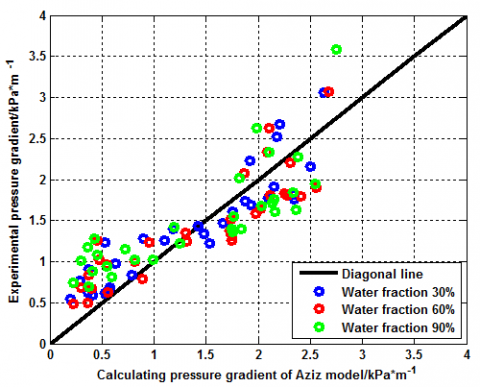Figure 1. Comparison results between the Aziz pressure gradient and experimental pressure gradient

With regard to various liquid flow rates, a curve similar to the abscissa GLR and the ordinate predicted residual is shown in Figure 2. In Figure 2, three results can be seen: (1) For the residual curves with water content 30%, 60% and 90%, there is no residual curve between the other two residual curves. This shows that there is no significant regularity between the pressure gradient and the water fraction; (2) As the liquid flow rate increases, the value of gas liquid ratio corresponding to the maximum prediction residual has a decreasing trend; (3) For the constant liquid flow rate, the prediction residual curves of gas liquid ratio have certain similarities in their geometry shapes. Hence, with respect to the constant liquid flow rate, this paper establishes the correlation between the prediction residual and the gas liquid ratio.

## figure_1a.png## figure_2b.png## figure_2c.png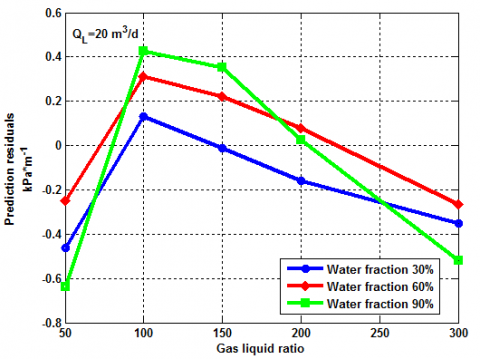## figure_2d.png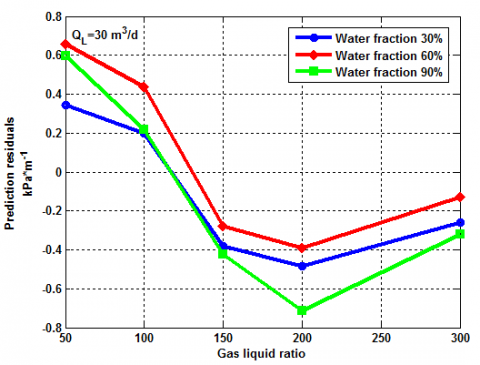## figure_2e.png## figure_2f.pngFigure 2. Correlation between the prediction residual and the gas liquid ratio

3. Cubic Function Fitting Model of Aziz Residual

Bases on the foregoing analysis, for six different liquid flow rates, the cubic polynomial function must be used to fit the prediction residual with respect to the gas liquid ratio. Because of the large difference in the orders of magnitude between the Aziz residual and the gas liquid ratio, before building the cubic function fitting, the gas liquid ratio is divided by 300.

This paper applies the cubic polynomial function to obtain the improved Aziz residual model, denoted as the Aziz-I model. It is shown in Eq(1).

$\hat{r}_{j}=A_{j}\left(\frac{z_{j}}{300}\right)^{3}+B_{j}\left(\frac{z_{j}}{300}\right)^{2}+C_{j}\left(\frac{z_{j}}{300}\right)+F_{j}$

$j=1,2, \cdots, 6$(1)

In Eq(1), $Z_{j}$ represents the gas liquid ratio with unit m3/m3; $\hat{r}_{j}$ is the fitting value of the prediction residual of the Aziz model with unit kPa/m; $A_{j}, B_{j}, C_{j},$ and $F_{j}$ for $j=1,2, \cdots, 6$ are undetermined fitting coefficients.

The values of undetermined coefficients $A_{j}, B_{j}, C_{j}$ and $F_{j}$ for $j=1,2, \cdots$,6 can be determined by the least square method [14-16] or some optimization algorithms [17-19].

In this paper, we use an improved particle swarm optimization , which is described in the next section, to determine the coefficients $A_{j}, B_{j}, C_{j},$ and $F_{j}$ for $j=1,2, \cdots, 6$

4. New Chaos Particle Swarm Optimization Combined with Chaotic Perturbation

The specific steps of the new chaos particle swarm optimisation algorithm combined with the chaotic perturbation are described as follows.

Step 1 Initialize the inertia weight $W_{\max } \quad$ and $\quad W_{\min }$, the learning factor $c_{1}$ and $c_{2}$, the group size $N$, the maximum iterations $\operatorname{MaxD} T$, the problem dimension $D$, and the accuracy control $e p s=10^{-6}$. Give the optimal space $[l b, u b]$ and the speed limit $v_{\max }$. Give the maximum iterations of chaos search $H D T$.

Step 2 Randomly produce a  $D$-dimensional particle over the interval $[0,1)$. According to the statement in section 2.2, obtain N s D-dimensional particles which are denoted as $x_{i}, i=1,2, \cdots, N$. A random D-dimensional space vector is selected from $[0,1)$. Use chaos mapping  to obtain $N-1$ s particles, and obtain the N s D-dimensional vector which is the initial particle speed. Let the iterations be 0, and continue to step 3.

Step 3 Insert $\mathcal{X}_{i}$ into the objective function to calculate the fitness  $f_{i}$, and determine the global optimal positions of the particle swarm gbest, the experienced optimal positions of particles $i=1,2, \cdots, N$. Continue to step 4.

Step 4 $\mathcal{W}$ decreases according to Eq(2). The positions and speed of the particles are updated according to Eq(3) and Eq(4). The iterations increase by 1. Take $k_{1}=\frac{M a x D T-t+1}{\operatorname{Max} D T}$, and determine the chaotic mutation and the chaos search interval $[l b(t), u b(t)]$ according to Eqs(5) and (6). Perform chaotic mutation to update pbest  and  pbest $_{i}$. Perform chaotic search to  pbest, and update pbest  and  pbest $_{i}$. Compute the fitness variance of the current particle swarm. If the absolute value of the difference between the fitness variance of the current particle swarm and that of the pre-iteration is less than $e p s$. Go to step 5, or step 6.

$w=w_{\max }-t \times\left(w_{\max }-w_{\min }\right) / \operatorname{Max} D T$(2)

\begin{aligned} v(t+1)=& w \times v(t)+c_{1} \times r a n d 1 \times(p b e s t-x(t)) \\ &+c_{2} \times r a n d 2 \times(g b e s t-x(t)) \end{aligned}(3)

$x(t+1)=x(t)+v(t+1)$(4)

$C D(t)=k_{1} \times k_{2} \times \cdots \times k_{t} \times C D(0)$(5)

$u b(t)=\left(1-k_{1} k_{2} \cdots k_{t}\right) \times x(t)+k_{1} k_{2} \cdots k_{t} \times u b$(6)

Step 5 Compute the numbers of the particles S that need to be replaced. Produce Ss new particles using a similar manipulation to produce the initial particle positions. Use the new particles to replace the current Ss particles that have the worst fitness. Then go to step 4.

Step 6 If the iterations are less than $\operatorname{Max} D T$, go to step 4. Otherwise, go to step 7.

Step 7 Output the final results: gbest and fbest.

For different liquid flow rates, the values of  $A_{j}, B_{j}, C_{j}$, and $F_{j}$ for $j=1,2, \cdots, 6$ are shown in Table 1.

Table 1. Values of undetermined coefficients for different liquid flow rates

 Liquid flow rate（m3/d） $A_{j}$ $B_{j}$ $C_{j}$ $F_{j}$ 10 -0.9011 -1.1512 3.6104 -1.1542 15 10.2898 -21.2416 12.7851 -1.9154 20 11.6489 -23.4322 13.4969 -2.0891 30 6.6078 -8.0887 0.6005 0.6505 40 -7.3502 16.3762 -11.0033 1.8583 50 -18.8094 35.8775 -19.9684 2.7399

When the liquid flow rates are taken as 10 m3/d, 30 m3/d and 50 m3/d respectively, the Aziz residual values and the improved Aziz residual values are shown in Figure 3.

In Figure 3, the black curve represents the improved Aziz residual curve by fitting. Figure 3 shows that the improved Aziz residual value and the Aziz residual are very close at the measure point set by the experimental scheme. Hence, it is feasible to use the improved Aziz residual curve to approximate the Aziz residual. The improved Aziz residual model can be taken as a reliable estimation model of the Aziz residual.

## figure_3a.png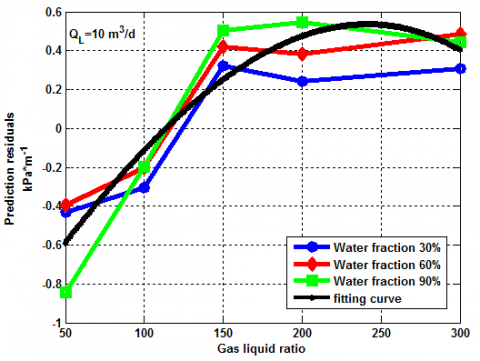## figure_3b.png## figure_3c.png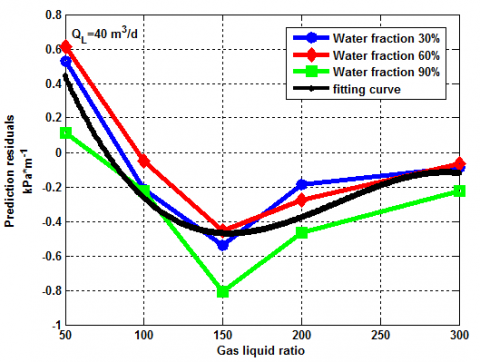Figure 3. Comparison between Aziz residual and the improved Aziz residual

5. Improved Aziz Model

As indicated in the introduction, the Aziz residual is obtained by subtracting the experimental pressure gradient from the Aziz pressure gradient. By combining the Aziz model and the improved Aziz residual model of residual, denoted as Aziz-RF model, the pressure gradient prediction model can be established, denoted as the Aziz-I model, which is obtained by subtracting the Aziz-RF model from the Aziz model.

The prediction pressure gradient of the Aziz-I model is

Aziz-IValue$=$Aziz Value-Aziz-RF Value(7)

where, Aziz-IValue denotes the calculating pressure gradient value from the Aziz-I model with unit kPa/m; Aziz Value denotes the calculating pressure gradient value from the Aziz model with unit kPa/m; $A z i z-R F$ Value denotes the improved Aziz residual value with unit kPa/m.

The relative error between the prediction pressure gradient of the Aziz model and the experimental pressure gradient is defined in Eq(10).

err$=\frac{ | A z i z-I \text { Value-E Value } |}{\text {EValue}}$(8)

In Eq(8), E Value denotes the experimental pressure gradient with unit kPa/m.

According to the 90 groups of experimental data shown in Figure 2, the average value of the prediction relative error of the Aziz-I model is 10.82% by comparing the relative error between the Aziz prediction pressure gradient and the experimental pressure gradient.

The comparison between the calculating pressure gradient of the Aziz-I model and the experimental pressure gradient is shown in Figure 4.

## figure_4.png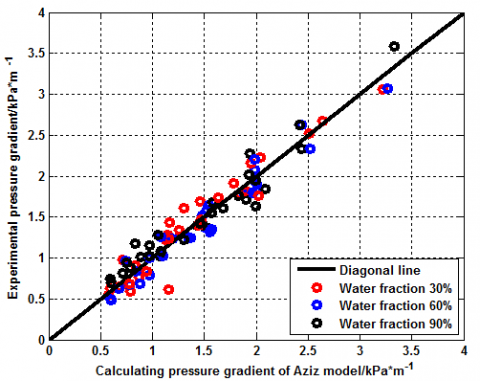Figure 4. Comparison between the calculating pressure gradient of the Aziz-I model and experimental pressure gradient

By comparing the results in Figure 1, the distribution of data points is closer to the diagonal. It shows that the new model (Aziz-I model) has higher accuracy.

6. Conclusions

(1) This paper firstly establishes the cubic fitting function model of the Aziz prediction residual with respect to the gas liquid ratio. Then, it builds a new pressure gradient prediction model (Aziz-I model) by combining the cubic fitting function model and the Aziz model.

(2) For the pressure gradient under the experimental condition, the average prediction relative error value of the Aziz-I model is 10.82%. This is a significant improvement on the prediction accuracy of the Aziz model.

(3) The Aziz-I model proposed in this paper is more concise compared to that in reference . It also has good extendibility.

Acknowledgment

The authors wish to thank the Branch of Key Laboratory of CNPC for Oil and Gas Production for their great help. This paper is supported by National Natural Science Foundation of China (61572084 and 51504038).

Nomenclature
 $A$ dimensionless fitting coefficient Aziz Value calculating pressure gradient value by Aziz model, kPa/m $A z i z-I$ Value calculating pressure gradient value by Aziz-I model, kPa/m $A z i z-R F$ Value improved Aziz residual value, kPa/m $B$ dimensionless fitting coefficient $C$ dimensionless fitting coefficient $\mathcal{C}_{1}$ learning factor $C_{2}$ learning factor $C D$ length of the corresponding interval of the mutation $D$ problem dimension $e p s$ accuracy control $E$ Value experimental pressure gradient, kPa/m $F$ dimensionless fitting coefficient $f_{i}$ fitness fbest optimum value gbest global optimal positions of the particle swarm $H D T$ maximum iterations of chaos search $k$ adjustment coefficient of weight $l b$ lower limit of the initial search space $u b$ upper limit of the initial search space $\operatorname{Max} D T$ maximum iterations $N$ group size pbest $_{i}$ experienced optimal positions of particles $r$ fitting value of the prediction residual of Aziz model, kPa/m rand 1 uniform random numbers over the interval [0,1] rand 2 uniform random numbers over the interval [0,1] $\boldsymbol{S}$ numbers of the particles which is needed to be replaced $t$ current iterations $\mathcal{V}_{\max }$ speed limit $\mathcal{V}$ particle velocity $\mathcal{W}$ weight $\mathcal{W}_{\max }$ maximum of the weight $\mathcal{W}_{\min }$ minimum of the weight $x$ particle position $\mathcal{X}_{\dot{i}}$ the i-th D-dimensional particle $Z$ gas liquid ratio, m3/m3 Subscripts $\dot{\imath}$ serial number of the particle $j$ the number of liquid flow rates
References

 A. S. Kaya, C. Sarica and J. P. Bril, “Mechanistic modeling of two-phase flow in deviated wells,” Spe Production and Facilities, vol. 16, no. 3, pp. 156–165, Aug., 2011. DOI: 10.2118/72998-PA.

 Tian Xianglei, Jiang Haiyan, Yue Yanru and Zhang Yongchang, “Research on production law for water drainage and gas production by gas lift,” Fault-Block Oil & Gas Field, vol. 19, no. 6, pp. 760–763, Feb., 2013. DOI: 10.6056/dkyqt201206020.

 Bai Xiaohong, Tian Wei, Tian Shubao and Li Kang, “Optimization on wellbore flow parameters of gas lift deliquification in low production and liquid loading gas wells,” Fault-Block Oil & Gas Field, vol. 21, no. 1, pp. 125–128, Jan. 2014. DOI: 10.6056/dkyqt201401031.

 Tian Yun, Wang Zhibin, Li Yingchuan, Bai Huifang and Li Kezhi, “Evaluation and optimization of wellbore pressure drop model for drainage and gas recovery by velocity string,” Fault-Block Oil & Gas Field, vol. 22, no. 1, pp. 130–133, Jan. 2015. DOI: 10.6056/dkyqt201501029.

 M. Attia, A. Abdulraheem and M. A. Mahmoud, “Pressure drop due to multiphase flow using four artificial intelligence methods,” in SPE North Africa Technical Conference and Exhibition, 2015.

 J. A. Ayoub and A. D. Hill, “Production operations R&D—Optimizing performance from the sandface to the tanks,” Journal of Petroleum Technology, vol. 56, no. 7, pp. 36–39, Jan. 2004.

 Mohsen Ebrahimi and Ali Sajedian, “Use of fuzzy logic for predicting two-phase inflow performance relationship of horizontal oil wells,” in Trinidad and Tobago Energy Resources Conference, 2010.

 Ahmed Al-Shammari. “Accurate prediction of pressure drop in two-phase vertical flow systems using artificial intelligence,” in SPE/DGS Saudi Arabia Section Technical Symposium and Exhibition, 2011.

 W  Al-Mudhafer,  M  Alabbas,  “Application  of  a  hybrid  system  of  genetic algorithm  &  fuzzy  logic  as  optimization  techniques for improving oil recovery  in  a  sandstone  reservoir  in  Iraq,” in SPE Latin America and Caribbean Petroleum Engineering Conference, 2012.

 M. Attia, A. Abdulraheem, M. A. Mahmoud, “Pressure drop due to multiphase flow using four artificial  intelligence  methods,”  in SPE  North  Africa  Technical Conference and Exhibition, 2015.

 K Aziz, GW Govier, “Pressure drop in wells producing oil and gas,” The Journal of Canadian Petroleum Technology, vol. 11, no. 3, pp. 38–48, July 1972. DOI: 10.2118/72-03-04.

 Mengxia Li, Ruiquan Liao, Yong Dong and Wei Luo, “New prediction method of pressure drop for oil-gas-water three-phase vertical flow,” J Balk Tribol AssoC, vol. 22, no. 1A-I, pp. 680-695, 2016.

 Yong Dong, Mengxia Li, Ruiquan Liao and Wei Luo, “Modification of Beggs-Brill pressure gradient predicting model for multiphase flow in vertical wells,” Journal of Oil and Gas Technology, vol. 38, no. 1, pp. 40–47, Mar. 2016. DOI: 10.12677/jogt.2016.11006.

 O. Axelsson, “A generalized conjugate gradient, least square method,” Numerische Mathematik, vol. 51, no. 2, pp. 209–227, Mar. 1987. DOI: 10.1007/BF01396750.

 Y. Shi, C. H. Liang, “The finite-volume time-domain algorithm using least square method in solving Maxwell’s equations,” Journal of Computational Physics, vol. 226, no. 2, pp. 1444-1457, Oct. 2007. DOI: 10.1016/j.jcp.2007.05.033.

 W. Zeng and S. Feng, “Approximate reasoning algorithm of interval-valued fuzzy sets based on least square method,” Information Sciences, vol. 272, no. 8, pp. 73–83, July 2014. DOI: 10.1016/j.ins.2014.02.078.

 Tao Lin, Peng Wu and Fengmei Gao, “Study on SVM temperature compensation of liquid ammonia volumetric flowmeter based on variable weight PSO,”  Int J Heat & Tech, vol. 33, no. 2, pp. 151–156, Jun. 2015. DOI: 10.18280/ijht.330224.

 C. H. Liang, S. Zeng, Z. X. Li, D. G. Yang and S. A. Sherif, “ Optimal design of plate-fin heat sink under natural convection using a particle swarm optimization algorithm,”  Int J Heat & Tech, vol. 34, no. 2, pp. 275-280, Jun. 2016. DOI: 10.18280/ijht.340217.

 Li Mengxia and Liao Ruiquan, “A new chaos particle swarm optimization combining the chaotic perturbation,” IJSIP, vol. 8, no. 4, pp. 41-48, April 2015. DOI: 10.14257/ijsip.2015.8.4.04.

 R. Anderson, “Industrial cryptography,” Iee Review, vol. 42, no. 3, pp. 118-120, May 1996. DOI: 10.1049/ir:19960314.